# Whole number exponents with integer bases

##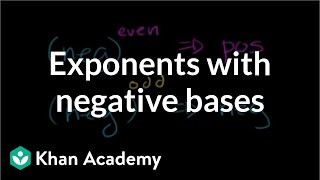By Khan Academy

Apply what we know about negative numbers to determine how negative bases with exponents are affected and what patterns develop. Also learn how order of operations affect the pattern.# Whole number exponents with integer bases

##By Khan Academy

Apply what we know about negative numbers to determine how negative bases with exponents are affected and what patterns develop. Also learn how order of operations affect the pattern.# Whole number exponents with integer bases

##By Khan Academy

Apply what we know about negative numbers to determine how negative bases with exponents are affected and what patterns develop. Also learn how order of operations affect the pattern.# Whole number exponents with integer bases

##By Khan Academy

Apply what we know about negative numbers to determine how negative bases with exponents are affected and what patterns develop. Also learn how order of operations affect the pattern.# Whole number exponents with integer bases 2

##By Khan Academy

Apply what we know about negative numbers to determine how negative bases with exponents are affected and what patterns develop. Also learn how order of operations affect the pattern.# Whole number exponents with integer bases

##By Khan Academy

Apply what we know about negative numbers to determine how negative bases with exponents are affected and what patterns develop. Also learn how order of operations affect the pattern.# Exponents with negative fractional bases

##By Khan Academy

Apply what we know about negative numbers to determine how negative bases with exponents are affected and what patterns develop. Also learn how order of operations affect the pattern.# Whole number exponents with integer bases 2

##By Khan Academy

Apply what we know about negative numbers to determine how negative bases with exponents are affected and what patterns develop. Also learn how order of operations affect the pattern.# Exponents with negative fractional bases

##By Khan Academy

Apply what we know about negative numbers to determine how negative bases with exponents are affected and what patterns develop. Also learn how order of operations affect the pattern.# Whole number exponents with integer bases 2

##By Khan Academy

Apply what we know about negative numbers to determine how negative bases with exponents are affected and what patterns develop. Also learn how order of operations affect the pattern.# Whole number exponents with integer bases 2

##By Khan Academy

Apply what we know about negative numbers to determine how negative bases with exponents are affected and what patterns develop. Also learn how order of operations affect the pattern.# Whole number exponents with integer bases 2

##By Khan Academy

Apply what we know about negative numbers to determine how negative bases with exponents are affected and what patterns develop. Also learn how order of operations affect the pattern.# Exponents with negative fractional bases

##By Khan Academy

Apply what we know about negative numbers to determine how negative bases with exponents are affected and what patterns develop. Also learn how order of operations affect the pattern.# Exponents with negative fractional bases

##By Khan Academy

Apply what we know about negative numbers to determine how negative bases with exponents are affected and what patterns develop. Also learn how order of operations affect the pattern.# Best tricks for squaring numbers 1-100 mentally! | Math tricks

##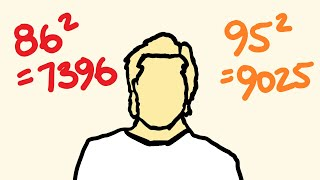By tecmath# Algebra Shortcuts - Work smarter and faster!

##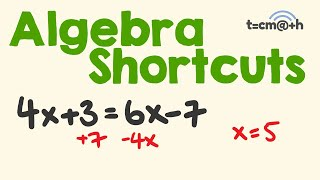By tecmath# How to convert between decimal and binary

##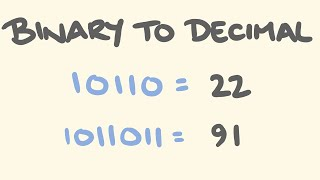By tecmath# 4th Grade Spatial Reasoning with Polygons

##By

4th grade math lesson on spatial reasoning with polygons, aligned to MA Curriculum Framework Standard 4.OA.5.# Calculate Exponents & Learn to Use Exponents in Math - [5-7-15]

##By Math and Science

Quality Math And Science Videos that feature step-by-step example problems!##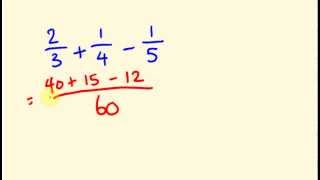By tecmath